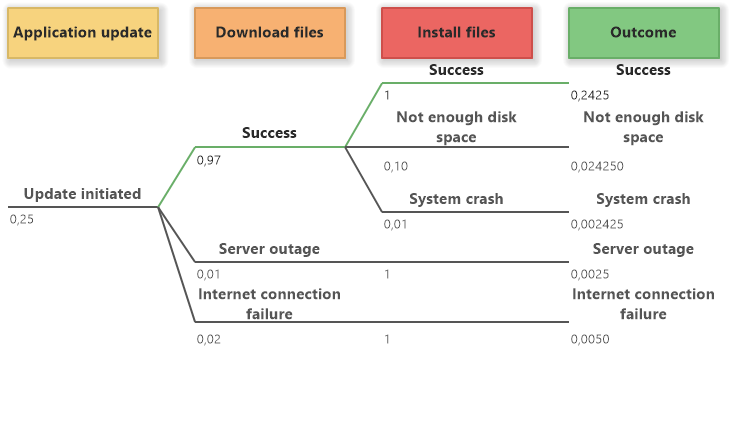# Event Tree Analysis (ETA)

An event tree analysis is a powerful analytical tool that allows you to analyze an event within a system with its possible consequences and probabilities of various outcomes.

## What is Event Tree Analysis?

An event tree analysis explores an initial event and its effects on the analyzed system. It shows an initial event and the events that may follow it with various options. The initial event is usually placed on the left and the outcomes on the right. The possible events are depicted in the middle. During the analysis, the tree of options is built and the probabilities for each option are identified and entered.

The result of the analysis is the outcome column that shows possible outcome options as a reaction to the initial event. Each outcome option has a calculated probability of its occurrence.

## Event Tree Analysis Symbols

An event tree analysis diagram offers the tools for the following elements:

• Initial Event - a lane of the event that initiates the whole process.
• Event - a lane for the following events and their options.
• Outcome - a lane for the outcome options with their calculated probabilities.
• Option - a scenario of the event.
• Outcome Option - a scenario of the outcome.
• Connector - connects an option with a following option.

## How to Create an Event Tree Analysis Diagram?

1. Drag Initial Event button from the Event Tree Analysis group in the toolbox into the diagram canvas. An initial event with a starting option will be added to the diagram.

2. Select the initial event element and click on the Add Event button in the context bar. The following event is added after the initial event.

3. Select the option in the initial event and click on the Add Option button in the context bar. An option will be added to the event that follows the initial event. Repeating this step, you can add any number of options that follow the selected option as a reaction to the event.

4. Add as many events and options to the diagram as you want.

5. Enter the probabilities of particular options into the field below the option name. 0 is for 0% probability, 1 is for 100 % probability. (e.g. probability 1:250 = 0,4 % should be entered as 0,004)

6. Select an event and click on Add Outcome button in the context bar. The outcome will be added after the last event. Then click on Update Outcome button in the context bar. The outcome options (one for each option from the last event) will be populated to the outcome element. The outcome probability will be calculated.ETA Diagram Editing

## Event Tree Analysis ExampleApplication Update (ETA Example)

Comment